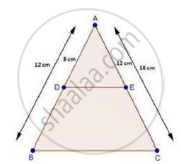Share

Books Shortlist
Your shortlist is empty

# Solution for In a δAbc, D and E Are Points on the Sides Ab and Ac Respectively. For the Following Case Show that De || Bc Ab = 2cm, Ad = 8cm, Ae = 12 Cm and Ac = L8cm. - CBSE Class 10 - Mathematics

ConceptBasic Proportionality Theorem Or Thales Theorem

#### Question

In a ΔABC, D and E are points on the sides AB and AC respectively. For  the following case show that DE || BC

AB = 2cm, AD = 8cm, AE = 12 cm and AC = l8cm.

#### SolutionAB = 12 cm, AD = 12 cm and AC = 18 cm.

∴ DB = AB – AD

= 12 – 8

⇒ DB = 4 cm

And, EC = AC – AE

= 18 – 12

⇒ EC = 6 cm

Now, "AD"/"DB"=8/4=2/1        [∵ DB = 4 cm]

And, "AE"/"EC"=12/6=2/1      [∵ EC = 6 cm]

rArr"AD"/"DB"="AE"/"EC"

Thus, DE divides sides AB and AC of ΔABC in the same ratio.

Therefore, by the converse of basic proportionality theorem,

We have, DE || BC

Is there an error in this question or solution?

#### Video TutorialsVIEW ALL 

Solution for question: In a δAbc, D and E Are Points on the Sides Ab and Ac Respectively. For the Following Case Show that De || Bc Ab = 2cm, Ad = 8cm, Ae = 12 Cm and Ac = L8cm. concept: Basic Proportionality Theorem Or Thales Theorem. For the course CBSE
S